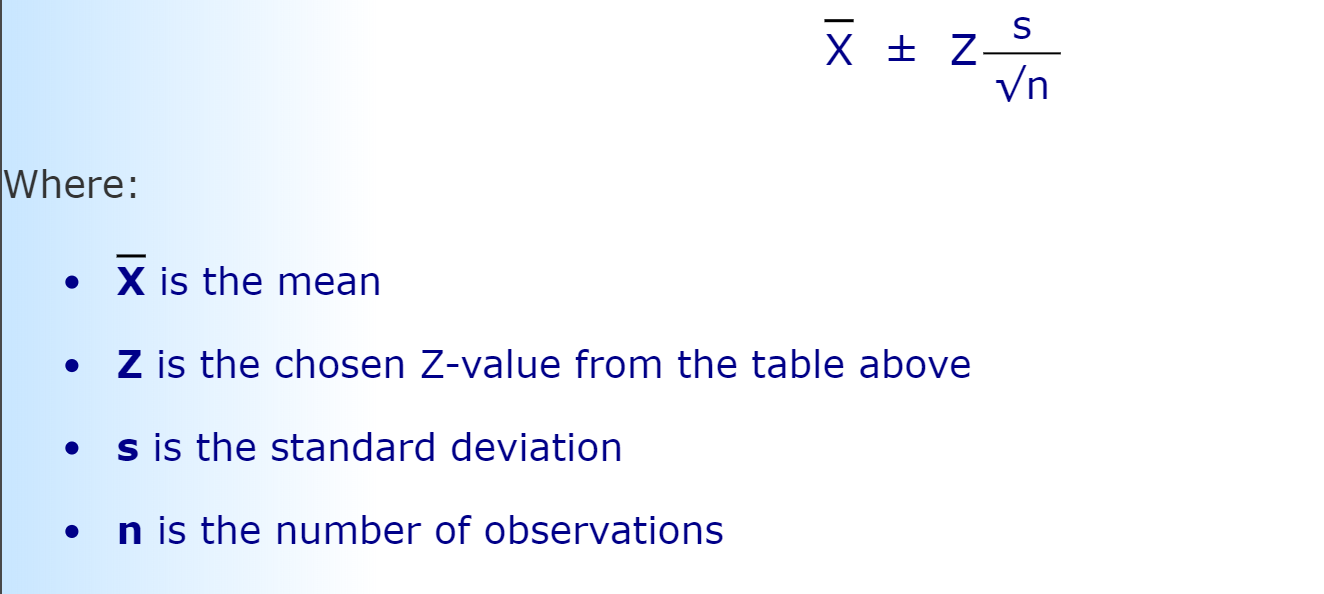## Programming Assignment 1: k-Nearest Neighbor Model for Binary Classification

#Programming-Assignment-1:-k-Nearest-Neighbor-Model-for-Binary-Classification
• Formula: @@0@@

Example:

• Formula: @@0@@

Example:

## 7. The accuracy and generalization error of two vectors

#7.-The-accuracy-and-generalization-error-of-two-vectors

Example:

## 8. Function: Precision, Recall and F1 Score

#8.-Function:-Precision,-Recall-and-F1-Score
• Precision scores: tp/(tp+fp). Intuitively, the precision scores tell out of all the correct prediction what percentage is the classifier correct true class and ground truth is true
• Recall scores: tp/(tp+fn). Intuitively, the recall scores tell the ability of classifier to find positve samples out of the data space
• F1 scores: weighted average of the precision and recall @@0@@

## Example (Comparing result with scikit function):

#Example-(Comparing-result-with-scikit-function):• True Positive(TP): predicted yes and ground truth = yes
• True Negative(TN): predicted no and ground truth = no
• False Positive(FP): predicted yes and ground truth = no
• False Negative(FN): predicted no and ground truth = yes
• NOTE: we try to match our implementation with sk_learn therefore, the order of the confusion matrix is as follow
1
2
3
4
Predicted 0   1
True
0         tn  fp
1         fn  tp
1
2
np.array([[tn, fp],
[fn, tp]])

Example:

• The receive operating characteristic (ROC) is a diagnotics tool as it probability thresh varies. This is a tool to select the best probability threshold for model when create a binary classification model.
• created by plotting the TPR and FPR at various thresh hold setting.
• True positive rate (TPR) @@0@@

• False positive rate (FPR): @@1@@

Example:

## 11. Compute area under curve (AUC) for the ROC curve

#11.-Compute-area-under-curve-(AUC)-for-the-ROC-curve

Example:

## 12. (BONUS) Function to generate the precision-recall curve

#12.-(BONUS)-Function-to-generate-the-precision-recall-curve

Example:

## Example (Check model performance on 'uniform' weight metric)

#Example-(Check-model-performance-on-'uniform'-weight-metric)

## Example (Check model performance on 'uniform' weight metric)

#Example-(Check-model-performance-on-'uniform'-weight-metric)

## Example (Sanity check the score board when y_labels is not given)

#Example-(Sanity-check-the-score-board-when-y_labels-is-not-given)

## 14. Read in white wine portion of the wine quality dataset from UCI's repository

• Use def = df.sample(frac=1)

## 20. Partition the data into train and test set

#20.-Partition-the-data-into-train-and-test-set

## 21. Naively run kNN model on train dataset with k=5 using L2

#21.-Naively-run-kNN-model-on-train-dataset-with-k=5-using-L2

## Define function to evaluate implemented and sklearn model

#Define-function-to-evaluate-implemented-and-sklearn-model

## 21a. Use Accuracy and F1 score to compare predictions to the expected variable

#21a.-Use-Accuracy-and-F1-score-to-compare-predictions-to-the-expected-variable

## Performance of implemented model (uniform weight) - unscaled

#Performance-of-implemented-model-(uniform-weight)---unscaled

## Performance of Scikit-learn model (uniform weight) - unscaled

#Performance-of-Scikit-learn-model-(uniform-weight)---unscaled

## 21b. Standardized data (subtract mean and divide by standard deviation)

#21b.-Standardized-data-(subtract-mean-and-divide-by-standard-deviation)

## 21c. Rerun the KNN model on the standardized data

#21c.-Rerun-the-KNN-model-on-the-standardized-data

## 21d. Compare the two accuracy values and the F1 scores

#21d.-Compare-the-two-accuracy-values-and-the-F1-scores

## Performance of implemented model (uniform weight) - scaled

#Performance-of-implemented-model-(uniform-weight)---scaled

## Performance of Scikit-learn model (uniform weight) - scaled

#Performance-of-Scikit-learn-model-(uniform-weight)---scaled
• After the comparison above, standardize data is used for the remainder of the data as it gives a better performance.

## 21e. Evaluate model with inverse distance weight metric

#21e.-Evaluate-model-with-inverse-distance-weight-metric

## Performance of implemented model (distance weight) - unscaled

#Performance-of-implemented-model-(distance-weight)---unscaled

## Performance of Sckit-learn model (distance weight) - unscaled

#Performance-of-Sckit-learn-model-(distance-weight)---unscaled

## Performance of implemented model (distance weight) - scaled

#Performance-of-implemented-model-(distance-weight)---scaled

## Performance of Sckit-learn model (distance weight) - scaled

#Performance-of-Sckit-learn-model-(distance-weight)---scaled

After the comparison above, distance weightage is preferred as it gives a better performance.

## 22. Implement K-fold(S-fold) cross validation function

#22.-Implement-K-fold(S-fold)-cross-validation-function

## 23. Use S-fold function to evaluate the performance of models

#23.-Use-S-fold-function-to-evaluate-the-performance-of-models

#23a.-k-=-

## Create performance table for report with respect to the different levels of neighbor and the different distance metrics

#Create-performance-table-for-report-with-respect-to-the-different-levels-of-neighbor-and-the-different-distance-metrics

## 23d. Determine the best model based on the overall performance (lowest average error)

#23d.-Determine-the-best-model-based-on-the-overall-performance-(lowest-average-error)